# Bicycle wheel

A bicycle wheel has a diameter of 60 cm. Approximately how many times does the wheel rotate at 2.5 km long trip?

N =  1326

### Step-by-step explanation: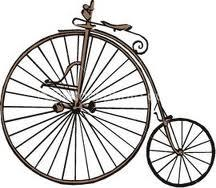Did you find an error or inaccuracy? Feel free to write us. Thank you!Tips to related online calculators
Do you want to convert length units?

#### You need to know the following knowledge to solve this word math problem:

We encourage you to watch this tutorial video on this math problem:

## Related math problems and questions:

• A bicycle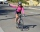A bicycle wheel has a diameter of 63 cm. Calculate how many times the wheel turns round in travelling 19.8 km .
• Bicycle wheel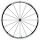Bicycle wheel diameter is 62 cm. How many times turns the bicycle on the road 1 km long?
• Wheel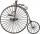Diameter of motocycle wheel is 52 cm. How many times rotates wheel on roand long 2 km?
• Velocipedes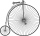In the 19th century, bicycles did not have a chain drive, and the wheel axis connected the pedals directly. This wheel diameter gradually increased until the so-called high bikes (velocipedes) with a front-wheel diameter of up to 1.5 meters, while the rea
• Wheel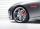How many times turns the wheel of a passenger car in one second if car run at speed 100 km/h. Wheel diameter is d = 62 cm.
• Wheels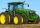Small tractor wheel with a diameter of 60 cm must be rotated 8 times to overcome some pathway. How many times must turn the big tractor wheel with a radius 60 cm to overcome the same distance?
• Wheel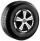What is the wheel diameter if on the 0.57 km track turns 106 times?
• GearsThe front gear on the bike has 32 teeth and the rear, on the wheel, has 12 teeth. How many times does the bike's rear-wheel turn if you turn the right pedal 30 times? What distance will you go if the circumference of the bicycle wheel is 250 cm?
• Diameter of a cylinderThe diameter of the cylinder is 42 cm. How many times does the cylinder turn on a 66m long track?
• Bicycle wheelAfter driving 157 m bicycle wheel rotates 100 times. What is the radius of the wheel in cm?
• Velocipede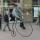The front wheel of velocipede from the year 1880 had a diameter 1.8 m. Suppose the front wheel turned again one, then the rear wheel 6 times. What was the diameter of the rear wheel?
• Earth's diameter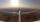The Earth's diameter on the equator is approximately 12750 km. How long does the Gripen fly over the Earth above the equator at 10 km if it is at an average speed of 1500 km / h?
• Pulley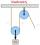On wheels with a diameter of 40 cm is fixed rope with the load. Calculate how far is load lifted when the wheel turns 7 times?
• Bicycle gears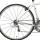The toothed wheel on the bicycle pedal has 40 teeth, the wheel on the rear wheel has only 16 teeth. How many times does the rear wheel turn if the pedals rotate 50 times?
• Odometer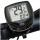The odometer is driven by the rotation of the wheel, whose diameter is 65 cm. After how many rotations, the wheel turns counter to the next kilometer?
• BaseBase of building is circle with diameter 25 m. Calculate the circumference of a circular trench witch diameter is 41 cm wider than the diameter of the base.
• Clock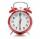What distance will pass end of 8 cm long hour hand for 15 minutes?